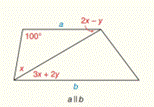Chapter 2.CR, Problem 14CR### Elementary Geometry for College St...

6th Edition
Daniel C. Alexander + 1 other
ISBN: 9781285195698

#### Solutions

Chapter
Section### Elementary Geometry for College St...

6th Edition
Daniel C. Alexander + 1 other
ISBN: 9781285195698
Textbook Problem
1 views

# For Review Exercises 12 to 15, find the values of x and y .To determine

To find:

The values of x and y.

Explanation

Given:

Line a is parallel to line b.

Figure (1)

Property:

(1) In a triangle the sum of all interior angles is 180°.

(2) If two parallel lines are cut by a transversal, the pair of two alternate interior angles is congruent.

Approach:

In ΔACD the sum of all interior angles is 180°.

So,

100+x+2xy=1803xy=1801003xy=80(1)

Line a is parallel to line b.

2xy and 3x+2y are alternate interior angles.

So,

### Still sussing out bartleby?

Check out a sample textbook solution.

See a sample solution

#### The Solution to Your Study Problems

Bartleby provides explanations to thousands of textbook problems written by our experts, many with advanced degrees!

Get Started

#### In Exercises 43-46, find f(a + h) f(a) for each function. Simplify yoiir answer. 45. f(x) = 4 x2

Applied Calculus for the Managerial, Life, and Social Sciences: A Brief Approach

#### In Problems 23-28, graph the functions.

Mathematical Applications for the Management, Life, and Social Sciences

#### Sometimes, Always, or Never: If for all n and both {an} and {cn} converge, then{bn} converges.

Study Guide for Stewart's Single Variable Calculus: Early Transcendentals, 8th